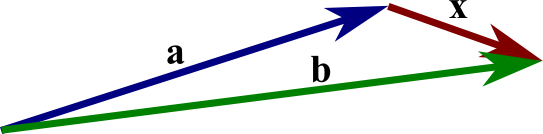# Math Insight

### Image: The difference of two vectorsThe difference of two vector $\vc{x}=\vc{b}-\vc{a}$ is formed by placing the tails of the two vectors together. Then, the vector $\vc{x}$ goes from the head of $\vc{a}$ to the tail of $\vc{b}$.

Image file: vector_b_minus_a.png

Source image file: vector_b_minus_a.svg
Source image type: Inkscape SVG